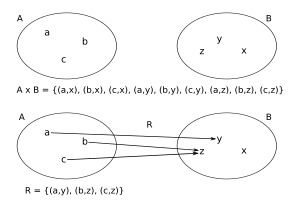# Relation (mathematics) facts for kids

Kids Encyclopedia FactsA relation "maps" elements of one set to another set. The example shows that several elements of the first set can be mapped to the same element of the second set. Both elements b and c of the first set map to element z of the second set.

In mathematics, a relation is used to describe certain properties of things. That way, certain things may be connected in some way; this is called a relation. It is clear, that things are either related, or they are not, there is no in-between. Relations are classified into four types based on mapping of elements.

Formally, a relation is a set of n-tuples of equal degree. Thus a binary relation is a set of pairs, a ternary relation a set of triples, and so forth. In the language of set theory, a relation between two sets is a subset of their Cartesian product.

The use of the term "relation" is often used as shorthand to refer to binary relations, where the set of all the starting points is called the domain and the set of the ending points is the range. The domain is the x's, and the range is the y's.

## Different types of relations

An example for such a relation might be a function. Functions associate keys with values. The set of all functions is a subset of the set of all relations - a function is a relation where the first value of every tuple is unique through the set.

Other well-known relations are the Equivalence relation and the Order relation. That way, sets of things can be ordered: Take the first element of a set, it is either equal to the element looked for, or there is an order relation that can be used to classify it. That way, the whole set can be classified (compared to some arbitrarily chosen element).

Relations can be transitive. One example of a transitive relation is "smaller-than". If X "is smaller than" Y, and Y is "smaller than" Z, then X "is smaller than" Z

Relations can be symmetric. One example of a symmetric relation is "is equal to". If X "is equal to" Y, Y "is equal to" X.

Relations can be reflexive. One example of a reflexive relation is "is equal to". X "is equal to" X.

Every subset of AxB is a relation from A to B.

Currying is a transformation that can be done to some relations: It is sometimes possible to change a relation that takes several arguments into a chain of relations that each only take one argument. Currying is often used in Lambda calculus.

## Databases and relations

Relationships are the established associations between two or more tables. Relationships are based on common fields from more than one table, often involving primary and foreign keys. See this article from MSDN: 

## Images for kidsRelation (mathematics) Facts for Kids. Kiddle Encyclopedia.